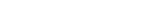MathExamle.com# Fraction

Select from the list a generator that creates examples with fraction answers and numbers

### Numerator

Creates examples with addition and subtraction of fractions, the answers are always positive

3 Variants 3 Levels
 5 5 + 35 + 612 = 8 8 41
Variant: 2 Level: Hard

Creates expressions with comparisons of fractions, the numbers are always positive

3 Variants 3 Levels
 473 < 74 59 10 150
Variant: A Level: Hard

### Denominator

Creates examples with addition and subtraction of fractions, the answers can be negative

3 Variants 3 Levels
 –44 10 + 43 1 = 23 2
 8 1 + 11 59 = 6 90
Variant: 1 Level: Hard

Creates examples with multiplication and division of fractions

3 Variants 3 Levels
 9 8 ÷ 5 = 13 26
 16 1 ÷ 99 = 2 26
Variant: 1 Level: Normal

Creates expressions with fractions which need to reduce

1 Variant 3 Levels
 171 = 45
 56 = 8
 2 = 8
Variant: 1 Level: Easy

Creates examples with operations of addition, subtraction, multiplication and division of fractions, the answers are always positive

2 Variants 3 Levels
 52 1 ÷ 315 – 60 1 = 2 404 3
 25 – 18 ÷ 696 = 116 29 35
Variant: 1 Level: Hard

Create expressions with comparisons of fractions, the numbers can be negative

3 Variants 3 Levels
 – 86480 < 3796 869 209
Variant: 1 Level: Hard

Creates examples with operations of addition, subtraction, multiplication and division of fractions, the answers can be negative

2 Variants 3 Levels
 9 ÷ 3 – 3 = 10 8 5
 – 1 × 17 + 8 1 = 2 5 2
Variant: 1 Level: Easy